#Numpy和Matplotlib

0
0
01. 云栖社区>
2. 博客>
3. 正文

## Numpy和Matplotlib

seancheney 2017-10-10 14:09:00 浏览902

# Python科学计算——Numpy

Numpy(Numerical Python extensions)是一个第三方的Python包，用于科学计算。这个库的前身是1995年就开始开发的一个用于数组运算的库。经过了长时间的发展，基本上成了绝大部分Python科学计算的基础包，当然也包括所有提供Python接口的深度学习框架。

array，也就是数组，是numpy中最基础的数据结构，最关键的属性是维度和元素类型，在numpy中，可以非常方便地创建各种不同类型的多维数组，并且执行一些基本基本操作，来看例子：

``````import numpy as np

a = [1, 2, 3, 4]        #
b = np.array(a)             # array([1, 2, 3, 4])
type(b)                     # <type 'numpy.ndarray'>

b.shape                     # (4,)
b.argmax()                  # 3
b.max()                     # 4
b.mean()                    # 2.5

c = [[1, 2], [3, 4]]    # 二维列表
d = np.array(c)             # 二维numpy数组
d.shape                     # (2, 2)
d.size                      # 4
d.max(axis=0)               # 找维度0，也就是最后一个维度上的最大值，array([3, 4])
d.max(axis=1)               # 找维度1，也就是倒数第二个维度上的最大值，array([2, 4])
d.mean(axis=0)              # 找维度0，也就是第一个维度上的均值，array([ 2.,  3.])
d.flatten()                 # 展开一个numpy数组为1维数组，array([1, 2, 3, 4])
np.ravel(c)               # 展开一个可以解析的结构为1维数组，array([1, 2, 3, 4])

# 3x3的浮点型2维数组，并且初始化所有元素值为1
e = np.ones((3, 3), dtype=np.float)

# 创建一个一维数组，元素值是把3重复4次，array([3, 3, 3, 3])
f = np.repeat(3, 4)

# 2x2x3的无符号8位整型3维数组，并且初始化所有元素值为0
g = np.zeros((2, 2, 3), dtype=np.uint8)
g.shape                    # (2, 2, 3)
h = g.astype(np.float)  # 用另一种类型表示

l = np.arange(10)       # 类似range，array([0, 1, 2, 3, 4, 5, 6, 7, 8, 9])
m = np.linspace(0, 6, 5)# 等差数列，0到6之间5个取值，array([ 0., 1.5, 3., 4.5, 6.])

p = np.array(
[[1, 2, 3, 4],
[5, 6, 7, 8]]
)

np.save('p.npy', p)     # 保存到文件
``````

``````import numpy as np

'''
array([[[ 0,  1,  2,  3],
[ 4,  5,  6,  7],
[ 8,  9, 10, 11]],

[[12, 13, 14, 15],
[16, 17, 18, 19],
[20, 21, 22, 23]]])
'''
a = np.arange(24).reshape((2, 3, 4))
b = a  # 17

'''
array([[ 8,  9, 10, 11],
[20, 21, 22, 23]])
'''
c = a[:, 2, :]

''' 用:表示当前维度上所有下标
array([[ 1,  5,  9],
[13, 17, 21]])
'''
d = a[:, :, 1]

''' 用...表示没有明确指出的维度
array([[ 1,  5,  9],
[13, 17, 21]])
'''
e = a[..., 1]

'''
array([[[ 5,  6],
[ 9, 10]],

[[17, 18],
[21, 22]]])
'''
f = a[:, 1:, 1:-1]

'''

[array([0, 1, 2]), array([3, 4, 5]), array([6, 7, 8])]
'''
g = np.split(np.arange(9), 3)

'''

[array([0, 1]), array([2, 3, 4, 5]), array([6, 7, 8])]
'''
h = np.split(np.arange(9), [2, -3])

l0 = np.arange(6).reshape((2, 3))
l1 = np.arange(6, 12).reshape((2, 3))

'''
vstack是指沿着纵轴拼接两个array，vertical
hstack是指沿着横轴拼接两个array，horizontal

stack不是拼接而是在输入array的基础上增加一个新的维度
'''
m = np.vstack((l0, l1))
p = np.hstack((l0, l1))
q = np.concatenate((l0, l1))
r = np.concatenate((l0, l1), axis=-1)
s = np.stack((l0, l1))

'''

array([[[ 0,  3],
[ 6,  9]],

[[ 1,  4],
[ 7, 10]],

[[ 2,  5],
[ 8, 11]]])
'''
t = s.transpose((2, 0, 1))

'''

array([[ 0,  4,  8],
[ 1,  5,  9],
[ 2,  6, 10],
[ 3,  7, 11]])
'''
u = a.transpose()    # 或者u=a.T也是获得转置

'''

array([[ 3,  2,  1,  0],
[ 7,  6,  5,  4],
[11, 10,  9,  8]])
'''
v = np.rot90(u, 3)

'''

array([[ 8,  4,  0],
[ 9,  5,  1],
[10,  6,  2],
[11,  7,  3]])
'''
w = np.fliplr(u)

'''

array([[ 3,  7, 11],
[ 2,  6, 10],
[ 1,  5,  9],
[ 0,  4,  8]])
'''
x = np.flipud(u)

'''

array([[11,  0,  4],
[ 8,  1,  5],
[ 9,  2,  6],
[10,  3,  7]])
'''
y = np.roll(u, 1)

'''

array([[ 8,  0,  4],
[ 9,  1,  5],
[10,  2,  6],
[11,  3,  7]])
'''
z = np.roll(u, 1, axis=1)
``````

``````import numpy as np

# 绝对值，1
a = np.abs(-1)

# sin函数，1.0
b = np.sin(np.pi/2)

# tanh逆函数，0.50000107157840523
c = np.arctanh(0.462118)

# e为底的指数函数，20.085536923187668
d = np.exp(3)

# 2的3次方，8
f = np.power(2, 3)

# 点积，1*3+2*4=11
g = np.dot([1, 2], [3, 4])

# 开方，5
h = np.sqrt(25)

# 求和，10
l = np.sum([1, 2, 3, 4])

# 平均值，5.5
m = np.mean([4, 5, 6, 7])

# 标准差，0.96824583655185426
p = np.std([1, 2, 3, 2, 1, 3, 2, 0])
``````

``````import numpy as np

a = np.array([
[1, 2, 3],
[4, 5, 6]
])

b = np.array([
[1, 2, 3],
[1, 2, 3]
])

'''

array([[2, 4, 6],
[5, 7, 9]])
'''
a + b

'''
array([[0, 0, 0],
[3, 3, 3]])
'''
a - b

'''
array([[ 1,  4,  9],
[ 4, 10, 18]])
'''
a * b

'''
array([[1, 1, 1],
[4, 2, 2]])
'''
a / b

'''
array([[ 1,  4,  9],
[16, 25, 36]])
'''
a ** 2

'''
array([[  1,   4,  27],
[  4,  25, 216]])
'''
a ** b

c = np.array([
[1, 2, 3],
[4, 5, 6],
[7, 8, 9],
[10, 11, 12]
])
d = np.array([2, 2, 2])

'''

d和c的每一行分别进行运算
array([[ 3,  4,  5],
[ 6,  7,  8],
[ 9, 10, 11],
[12, 13, 14]])
'''
c + d

'''
array([[ 2,  4,  6],
[ 8, 10, 12],
[14, 16, 18],
[20, 22, 24]])
'''
c * d

'''
1和c的每个元素分别进行运算
array([[ 0,  1,  2],
[ 3,  4,  5],
[ 6,  7,  8],
[ 9, 10, 11]])
'''
c - 1
``````

# 线性代数模块（linalg）

``````import numpy as np

a = np.array([3, 4])
np.linalg.norm(a)

b = np.array([
[1, 2, 3],
[4, 5, 6],
[7, 8, 9]
])
c = np.array([1, 0, 1])

# 矩阵和向量之间的乘法
np.dot(b, c)                    # array([ 4, 10, 16])
np.dot(c, b.T)                  # array([ 4, 10, 16])

np.trace(b)                     # 求矩阵的迹，15
np.linalg.det(b)                # 求矩阵的行列式值，0
np.linalg.matrix_rank(b)    # 求矩阵的秩，2，不满秩，因为行与行之间等差

d = np.array([
[2, 1],
[1, 2]
])

'''

array([[ 0.70710678, -0.70710678],
[ 0.70710678,  0.70710678]])

eig()是一般情况的本征值分解，对于更常见的对称实数矩阵，
eigh()更快且更稳定，不过输出的值的顺序和eig()是相反的
'''
u, v = np.linalg.eig(d)

# Cholesky分解并重建
l = np.linalg.cholesky(d)

'''
array([[ 2.,  1.],
[ 1.,  2.]])
'''
np.dot(l, l.T)

e = np.array([
[1, 2],
[3, 4]
])

# 对不镇定矩阵，进行SVD分解并重建
U, s, V = np.linalg.svd(e)

S = np.array([
[s, 0],
[0, s]
])

'''
array([[ 1.,  2.],
[ 3.,  4.]])
'''
np.dot(U, np.dot(S, V))
``````

# 随机模块（random）

``````import numpy as np
import numpy.random as random

# 设置随机数种子
random.seed(42)

# 产生一个1x3，[0,1)之间的浮点型随机数
# array([[ 0.37454012,  0.95071431,  0.73199394]])
# 后面的例子就不在注释中给出具体结果了
random.rand(1, 3)

# 产生一个[0,1)之间的浮点型随机数
random.random()

# 下边4个没有区别，都是按照指定大小产生[0,1)之间的浮点型随机数array，不Pythonic…
random.random((3, 3))
random.sample((3, 3))
random.random_sample((3, 3))
random.ranf((3, 3))

# 产生10个[1,6)之间的浮点型随机数
5*random.random(10) + 1
random.uniform(1, 6, 10)

# 产生10个[1,6)之间的整型随机数
random.randint(1, 6, 10)

# 产生2x5的标准正态分布样本
random.normal(size=(5, 2))

# 产生5个，n=5，p=0.5的二项分布样本
random.binomial(n=5, p=0.5, size=5)

a = np.arange(10)

# 从a中有回放的随机采样7个
random.choice(a, 7)

# 从a中无回放的随机采样7个
random.choice(a, 7, replace=False)

# 对a进行乱序并返回一个新的array
b = random.permutation(a)

# 对a进行in-place乱序
random.shuffle(a)

# 生成一个长度为9的随机bytes序列并作为str返回
# '\x96\x9d\xd1?\xe6\x18\xbb\x9a\xec'
random.bytes(9)
``````

``````import numpy.random as random

random.seed(42)

# 做10000次实验
n_tests = 10000

# 生成每次实验的奖品所在的门的编号
# 0表示第一扇门，1表示第二扇门，2表示第三扇门
winning_doors = random.randint(0, 3, n_tests)

# 记录如果换门的中奖次数
change_mind_wins = 0

# 记录如果坚持的中奖次数
insist_wins = 0

# winning_door就是获胜门的编号
for winning_door in winning_doors:

# 随机挑了一扇门
first_try = random.randint(0, 3)

# 其他门的编号
remaining_choices = [i for i in range(3) if i != first_try]

# 没有奖品的门的编号，这个信息只有主持人知道
wrong_choices = [i for i in range(3) if i != winning_door]

# 一开始选择的门主持人没法打开，所以从主持人可以打开的门中剔除
if first_try in wrong_choices:
wrong_choices.remove(first_try)

# 这时wrong_choices变量就是主持人可以打开的门的编号
# 注意此时如果一开始选择正确，则可以打开的门是两扇，主持人随便开一扇门
# 如果一开始选到了空门，则主持人只能打开剩下一扇空门
screened_out = random.choice(wrong_choices)
remaining_choices.remove(screened_out)

# 所以虽然代码写了好些行，如果策略固定的话，
# 改变主意的获胜概率就是一开始选错的概率，是2/3
# 而坚持选择的获胜概率就是一开始就选对的概率，是1/3

# 现在除了一开始选择的编号，和主持人帮助剔除的错误编号，只剩下一扇门
# 如果要改变注意则这扇门就是最终的选择
changed_mind_try = remaining_choices

# 结果揭晓，记录下来
change_mind_wins += 1 if changed_mind_try == winning_door else 0
insist_wins += 1 if first_try == winning_door else 0

# 输出10000次测试的最终结果，和推导的结果差不多：
# You win 6616 out of 10000 tests if you changed your mind
# You win 3384 out of 10000 tests if you insist on the initial choice
print(
'You win {1} out of {0} tests if you changed your mind\n'
'You win {2} out of {0} tests if you insist on the initial choice'.format(
n_tests, change_mind_wins, insist_wins
)
)
``````

# Python的可视化包 – Matplotlib

Matplotlib是Python中最常用的可视化工具之一，可以非常方便地创建海量类型地2D图表和一些基本的3D图表。Matplotlib最早是为了可视化癫痫病人的脑皮层电图相关的信号而研发，因为在函数的设计上参考了MATLAB，所以叫做Matplotlib。

# 2D图表

Matplotlib中最基础的模块是pyplot。先从最简单的点图和线图开始，比如我们有一组数据，还有一个拟合模型，通过下面的代码图来可视化：

``````import numpy as np
import matplotlib as mpl
import matplotlib.pyplot as plt

# 通过rcParams设置全局横纵轴字体大小
mpl.rcParams['xtick.labelsize'] = 24
mpl.rcParams['ytick.labelsize'] = 24

np.random.seed(42)

# x轴的采样点
x = np.linspace(0, 5, 100)

# 通过下面曲线加上噪声生成数据，所以拟合模型就用y了……
y = 2*np.sin(x) + 0.3*x**2
y_data = y + np.random.normal(scale=0.3, size=100)

# figure()指定图表名称
plt.figure('data')

# '.'标明画散点图，每个散点的形状是个圆
plt.plot(x, y_data, '.')

# 画模型的图，plot函数默认画连线图
plt.figure('model')
plt.plot(x, y)

# 两个图画一起
plt.figure('data & model')

# 通过'k'指定线的颜色，lw指定线的宽度
# 第三个参数除了颜色也可以指定线形，比如'r--'表示红色虚线
# 更多属性可以参考官网：http://matplotlib.org/api/pyplot_api.html
plt.plot(x, y, 'k', lw=3)

# scatter可以更容易地生成散点图
plt.scatter(x, y_data)

# 将当前figure的图保存到文件result.png
plt.savefig('result.png')

# 一定要加上这句才能让画好的图显示在屏幕上
plt.show()
``````

matplotlib和pyplot的惯用别名分别是mpl和plt，上面代码生成的图像如下：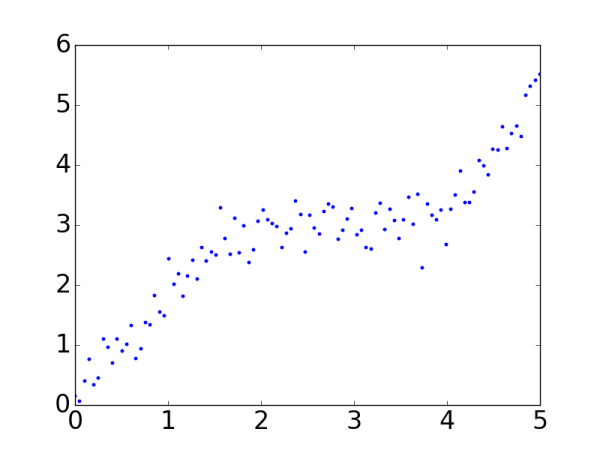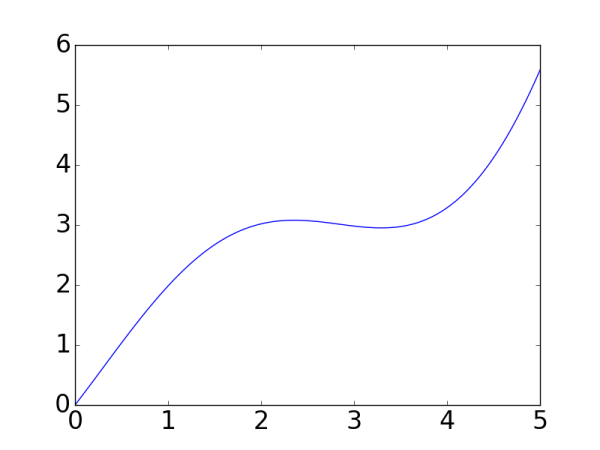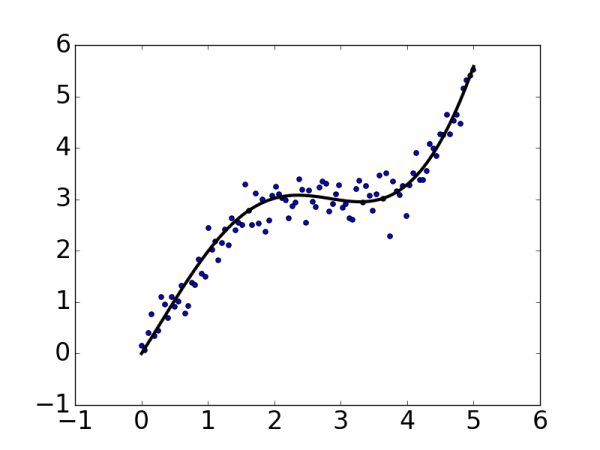pyplot - Matplotlib 1.5.3 documentation**
Customizing matplotlib**

``````import numpy as np
import matplotlib as mpl
import matplotlib.pyplot as plt

mpl.rcParams['axes.titlesize'] = 20
mpl.rcParams['xtick.labelsize'] = 16
mpl.rcParams['ytick.labelsize'] = 16
mpl.rcParams['axes.labelsize'] = 16
mpl.rcParams['xtick.major.size'] = 0
mpl.rcParams['ytick.major.size'] = 0

# 包含了狗，猫和猎豹的最高奔跑速度，还有对应的可视化颜色
speed_map = {
'dog': (48, '#7199cf'),
'cat': (45, '#4fc4aa'),
'cheetah': (120, '#e1a7a2')
}

# 整体图的标题
fig = plt.figure('Bar chart & Pie chart')

# 在整张图上加入一个子图，121的意思是在一个1行2列的子图中的第一张
ax.set_title('Running speed - bar chart')

# 生成x轴每个元素的位置
xticks = np.arange(3)

# 定义柱状图每个柱的宽度
bar_width = 0.5

# 动物名称
animals = speed_map.keys()

# 奔跑速度
speeds = [x for x in speed_map.values()]

# 对应颜色
colors = [x for x in speed_map.values()]

# 画柱状图，横轴是动物标签的位置，纵轴是速度，定义柱的宽度，同时设置柱的边缘为透明
bars = ax.bar(xticks, speeds, width=bar_width, edgecolor='none')

# 设置y轴的标题
ax.set_ylabel('Speed(km/h)')

# x轴每个标签的具体位置，设置为每个柱的中央
ax.set_xticks(xticks+bar_width/2)

# 设置每个标签的名字
ax.set_xticklabels(animals)

# 设置x轴的范围
ax.set_xlim([bar_width/2-0.5, 3-bar_width/2])

# 设置y轴的范围
ax.set_ylim([0, 125])

# 给每个bar分配指定的颜色
for bar, color in zip(bars, colors):
bar.set_color(color)

# 在122位置加入新的图
ax.set_title('Running speed - pie chart')

# 生成同时包含名称和速度的标签
labels = ['{}\n{} km/h'.format(animal, speed) for animal, speed in zip(animals, speeds)]

# 画饼状图，并指定标签和对应颜色
ax.pie(speeds, labels=labels, colors=colors)

plt.show()
``````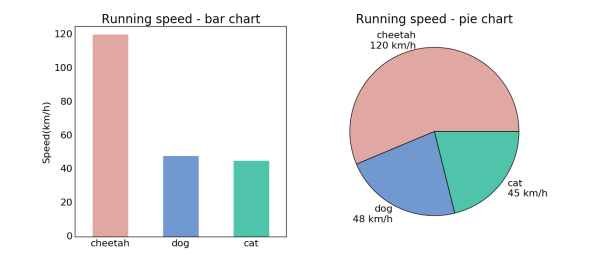# 3D图表

Matplotlib中也能支持一些基础的3D图表，比如曲面图，散点图和柱状图。这些3D图表需要使用mpl_toolkits模块，先来看一个简单的曲面图的例子：

``````import matplotlib.pyplot as plt
import numpy as np

# 3D图标必须的模块，project='3d'的定义
from mpl_toolkits.mplot3d import Axes3D

np.random.seed(42)

n_grids = 51            # x-y平面的格点数
c = n_grids / 2         # 中心位置
nf = 2                  # 低频成分的个数

# 生成格点
x = np.linspace(0, 1, n_grids)
y = np.linspace(0, 1, n_grids)

# x和y是长度为n_grids的array
# meshgrid会把x和y组合成n_grids*n_grids的array，X和Y对应位置就是所有格点的坐标
X, Y = np.meshgrid(x, y)

# 生成一个0值的傅里叶谱
spectrum = np.zeros((n_grids, n_grids), dtype=np.complex)

# 生成一段噪音，长度是(2*nf+1)**2/2
noise = [np.complex(x, y) for x, y in np.random.uniform(-1,1,((2*nf+1)**2/2, 2))]

# 傅里叶频谱的每一项和其共轭关于中心对称
noisy_block = np.concatenate((noise, [0j], np.conjugate(noise[::-1])))

# 将生成的频谱作为低频成分
spectrum[c-nf:c+nf+1, c-nf:c+nf+1] = noisy_block.reshape((2*nf+1, 2*nf+1))

# 进行反傅里叶变换
Z = np.real(np.fft.ifft2(np.fft.ifftshift(spectrum)))

# 创建图表
fig = plt.figure('3D surface & wire')

# 第一个子图，surface图
ax = fig.add_subplot(1, 2, 1, projection='3d')

# alpha定义透明度，cmap是color map
# rstride和cstride是两个方向上的采样，越小越精细，lw是线宽
ax.plot_surface(X, Y, Z, alpha=0.7, cmap='jet', rstride=1, cstride=1, lw=0)

# 第二个子图，网线图
ax = fig.add_subplot(1, 2, 2, projection='3d')
ax.plot_wireframe(X, Y, Z, rstride=3, cstride=3, lw=0.5)

plt.show()
``````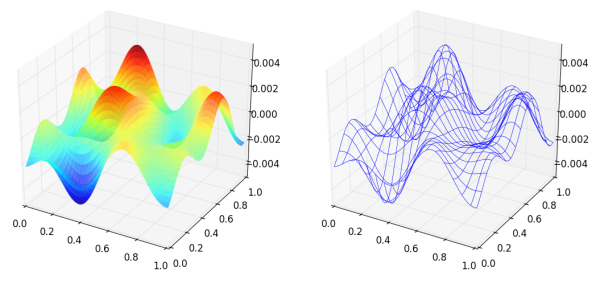3D的散点图也是常常用来查看空间样本分布的一种手段，并且画起来比表面图和网线图更加简单，来看例子：

``````import matplotlib.pyplot as plt
import numpy as np

from mpl_toolkits.mplot3d import Axes3D

np.random.seed(42)

# 采样个数500
n_samples = 500
dim = 3

# 先生成一组3维正态分布数据，数据方向完全随机
samples = np.random.multivariate_normal(
np.zeros(dim),
np.eye(dim),
n_samples
)

# 通过把每个样本到原点距离和均匀分布吻合得到球体内均匀分布的样本
for i in range(samples.shape):
r = np.power(np.random.random(), 1.0/3.0)
samples[i] *= r / np.linalg.norm(samples[i])

upper_samples = []
lower_samples = []

for x, y, z in samples:
# 3x+2y-z=1作为判别平面
if z > 3*x + 2*y - 1:
upper_samples.append((x, y, z))
else:
lower_samples.append((x, y, z))

fig = plt.figure('3D scatter plot')

uppers = np.array(upper_samples)
lowers = np.array(lower_samples)

# 用不同颜色不同形状的图标表示平面上下的样本
# 判别平面上半部分为红色圆点，下半部分为绿色三角
ax.scatter(uppers[:, 0], uppers[:, 1], uppers[:, 2], c='r', marker='o')
ax.scatter(lowers[:, 0], lowers[:, 1], lowers[:, 2], c='g', marker='^')

plt.show()
``````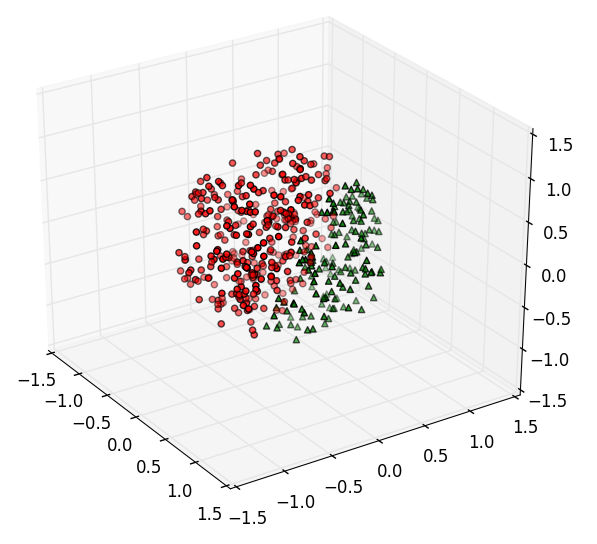# 图像显示

Matplotlib也支持图像的存取和显示，并且和OpenCV一类的接口比起来，对于一般的二维矩阵的可视化要方便很多，来看例子：

``````import matplotlib.pyplot as plt

# 读取一张小白狗的照片并显示
plt.figure('A Little White Dog')
plt.imshow(little_dog_img)

# Z是上小节生成的随机图案，img0就是Z，img1是Z做了个简单的变换
img0 = Z
img1 = 3*Z + 4

# cmap指定为'gray'用来显示灰度图
fig = plt.figure('Auto Normalized Visualization')
ax0.imshow(img0, cmap='gray')

ax1.imshow(img1, cmap='gray')

plt.show()
``````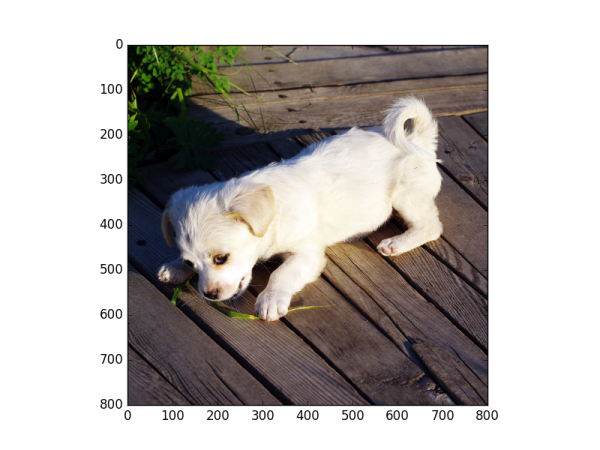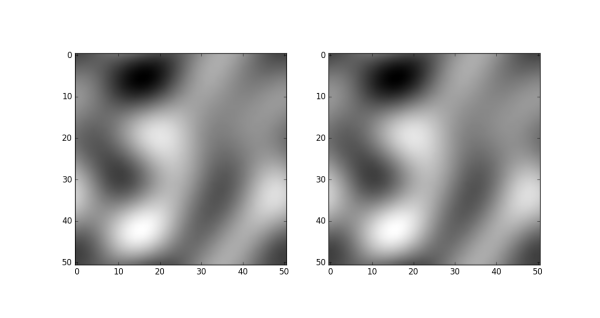Thumbnail gallery - Matplotlib 1.5.3 documentation

seancheney
+ 关注